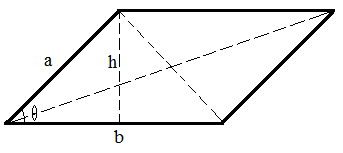## Parallelogram Calculator

 Base a: Base b: Altitude (h): Angle (θ): radian  ̊ degree  π  π/3 Perimeter: Area: Long Diagonal: Short Diagonal:

In geometry, a parallelogram with two pairs of parallel sides.### Parallelogram formula

1. Perimeter = 2a + 2b

2. Area = bh = absinθ

3. Long Diagonal = sqrt(a2 + b2 - 2abcos(π - θ));

4. Short Diagonal = sqrt(a2 + b2 - 2abcos(θ));

For example, when base a = 3, base b = 4, altitude(h) = 1, then the Angle (θ) = 0.3398, perimeter = 14, area = 2, Long Diagonal = 6.9013, Short Diagonal = 1.5403.

For example, when base a = 12, base b = 35, Angle (θ) = 33, then the Altitude (h) = 11.9989, perimeter = 94, area = 209.9808, Long Diagonal = 36.849, Short Diagonal = 37.1504.

Thinkcalculator.com provides you helpful and handy calculator resources.